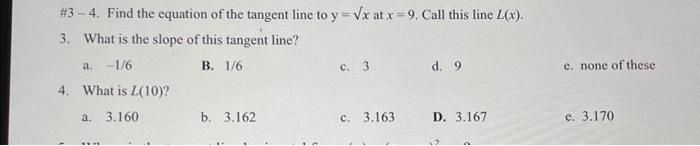Home / Expert Answers / Calculus / 34-find-the-equation-of-the-tangent-line-to-y-x-at-x-9-call-this-line-l-x-3-what-is-the-pa418

# (Solved): #34. Find the equation of the tangent line to y=x at x=9. Call this line L(x). 3. What is the .... Find the equation of the tangent line to at . Call this line . 3. What is the slope of this tangent line? a. B. c. 3 d. 9 e. none of these 4. What is ? a. 3.160 b. 3.162 c. 3.163 D. 3.167 e. 3.170

We have an Answer from Expert